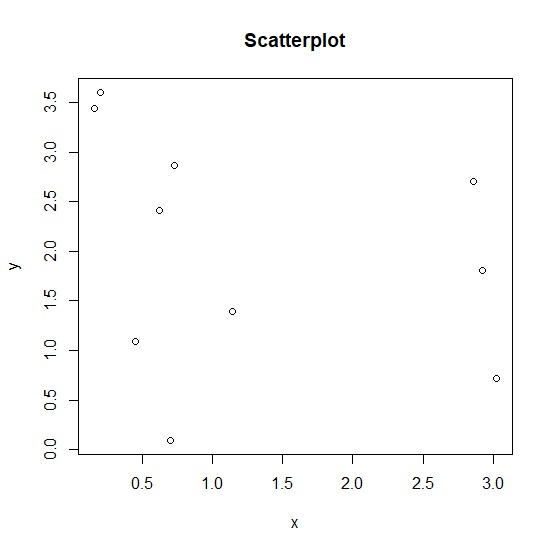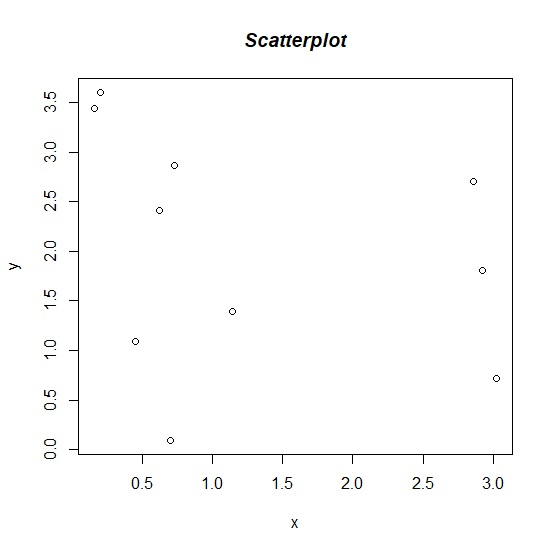# How to change the title of a graph to italic created by using plot function in R?

If a graph is created by specifying main title of the plot using the plot function then the default font is plain text. We might want to change the style of the font to italic so that the title gets a little more attraction of the viewers. This can be done by using font.main argument with plot function. The value 4 of font.main refers to the bold italic font and if we want to make it bold then we can use the value 3.

Consider the below vectors and create the scatterplot between the two with title of the plot −

## Example

x<-rnorm(10,2,1.5)
y<-rnorm(10,2,1) plot(x,y,main="Scatterplot")

## OutputCreating the same plot with title in italics −

## Example

plot(x,y,main="Scatterplot",font.main=4)

## output